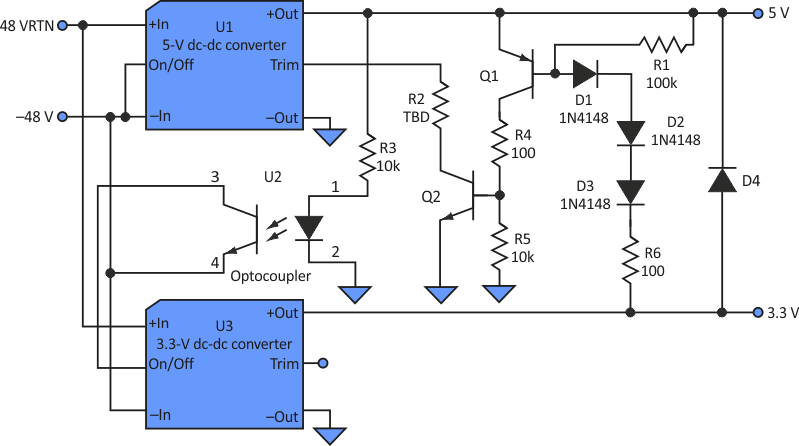Add two components to power supply to ensure proper voltage sequencing

Today's digital ICs typically demand complex voltage sequencing, a task that usually requires dedicated ICs or microprocessors. But what if your requirements are more modest? The sequencing scheme presented here requires only a single optocoupler and a resistor.

Assume that the dc-dc converter's On/Off pin is pulled low to turn on and floats to remain off (see the Fig. 1). Also, in this example, the 5 V needs to turn on before the 3.3 V. The 5-V converter's On/Off pin is tied low. The 3.3 V converter's On/Off pin remains floating when U2 is off.Figure 1. Adding an optocoupler (U2) and a resistor to this power circuit is all that’s needed to ensure the proper startup sequence of the 3.3- and 5-V converters.

When power is applied, the 5-V converter turns on. As the 5 V rises, the optocoupler eventually will be driven on, turning on the 3.3-V converter. (For a non-isolated application, the optocoupler could be replaced with a transistor.)

Many logic ICs that use multiple voltages limit the allowable difference in voltage between the 5 V and 3.3 V to around 2.5 V. Using this scheme, that limit could be exceeded if U1 reaches 5 V before the 3.3 V starts up, or if the 3.3 V fails. Let's assume the outputs of the dc-dc converters change in the direction that the converter's trim pin is pulled. Many converters spec the trim to be ±5%, but often the output can be pulled lower by the trim pin.

The difference voltage is limited when Q1's base emitter, D1, D2, and D3 conduct. Q1 then turns on, turning on Q2, which then pulls the trim pin down. Therefore, the 5-V output will regulate from 2 V to 2.5 V above the 3.3-V output. The actual regulation voltage will depend on where the junctions conduct. R2 is determined based on the chosen converter.

The final requirement is preventing the 5-V output from going more than 0.7 V below the 3.3-V output, which could happen at turn-off. Avoid this problem by using a Schottky diode for D4. The use of D4 isn't new, but is mentioned just for completeness.

If there's no voltage difference requirement but the 3.3 V should not turn on until the 5-V output reaches within 10% to 15% of its output, adding diodes or a Zener in series with R3 will work.

electronicdesign.com